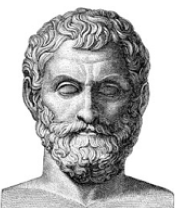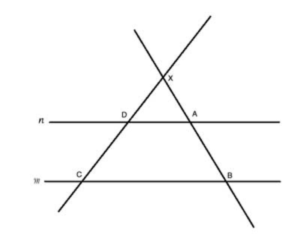## Lorem ipsum dolorThales of Miletus was a Greek mathematician, astronomer and pre-Socratic philosopher from Miletus in Ionia, Asia Minor. He was one of the Seven Sages of Greece.

In mathematics, Thales used geometry to calculate the heights of pyramids and the distance of ships from the shore. He is the first known individual to use deductive reasoning applied to geometry, by deriving four corollaries to Thales’ theorem.Thales’s therom
Thales’s theorem states that if A, B, and C are distinct points on a circle where the line AC is a diameter, then the angle ∠ABC is a right angle.Intercept therom
The Intercept theorem provides the ratios between the line segments created when two parallel lines are intercepted by two intersecting lines.

It is sometimes called “Thales’ Theorem”  (not to be confused with another one of his theorems related to inscribed angles)

It states that if m and n are parallel lines. XC and XB are two intersecting lines that intercept m and n. Show that the following relationship between the lengths of the line segments is true:
XD/XC=XA/XB =DA/CB and XD/DC=XA/AB